Скачать презентацию Statistical Inference Confidence Intervals and Hypothesis Testing —

9757dc729547934f1f59d89518018788.ppt

• Количество слайдов: 55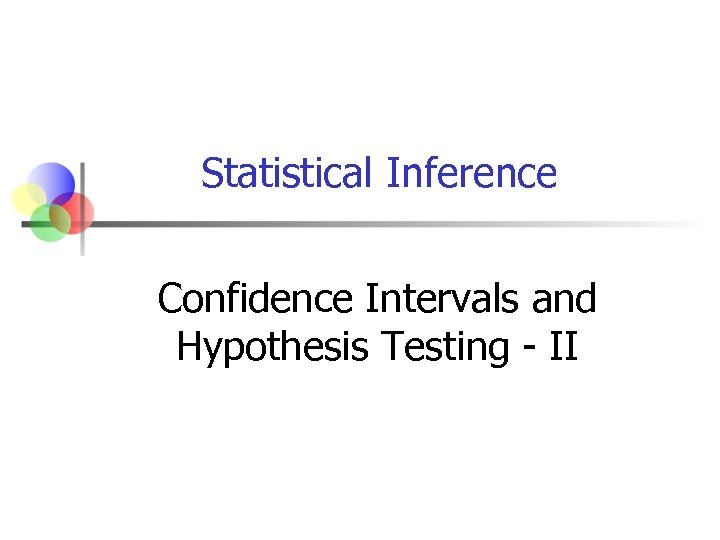Statistical Inference Confidence Intervals and Hypothesis Testing - II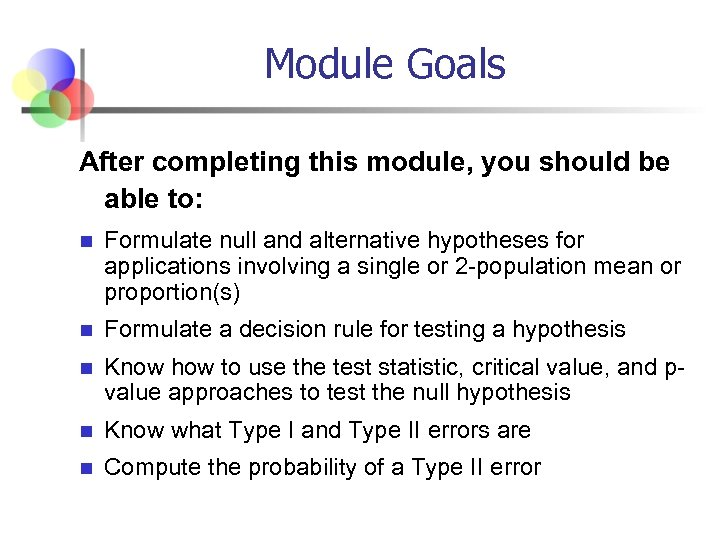Module Goals After completing this module, you should be able to: n Formulate null and alternative hypotheses for applications involving a single or 2 -population mean or proportion(s) n Formulate a decision rule for testing a hypothesis n Know how to use the test statistic, critical value, and pvalue approaches to test the null hypothesis n Know what Type I and Type II errors are n Compute the probability of a Type II error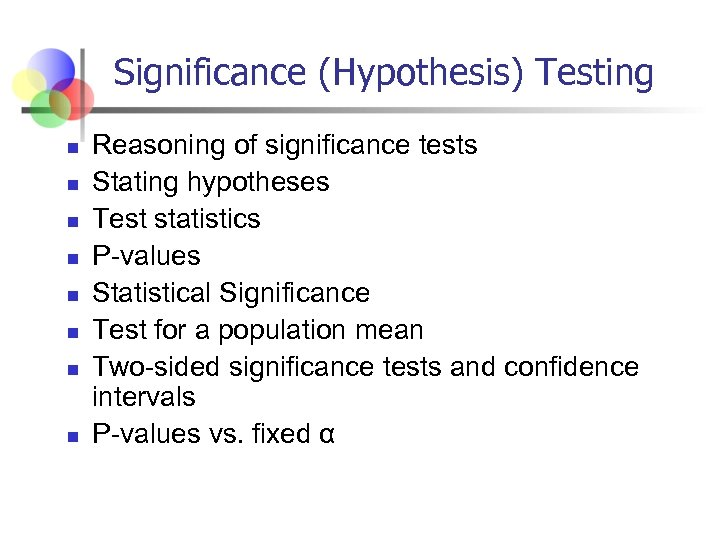Significance (Hypothesis) Testing n n n n Reasoning of significance tests Stating hypotheses Test statistics P-values Statistical Significance Test for a population mean Two-sided significance tests and confidence intervals P-values vs. fixed α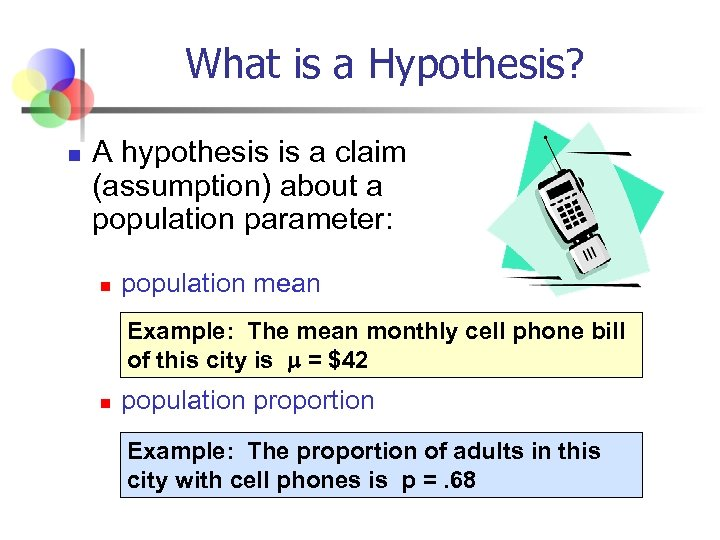What is a Hypothesis? n A hypothesis is a claim (assumption) about a population parameter: n population mean Example: The mean monthly cell phone bill of this city is = \$42 n population proportion Example: The proportion of adults in this city with cell phones is p =. 68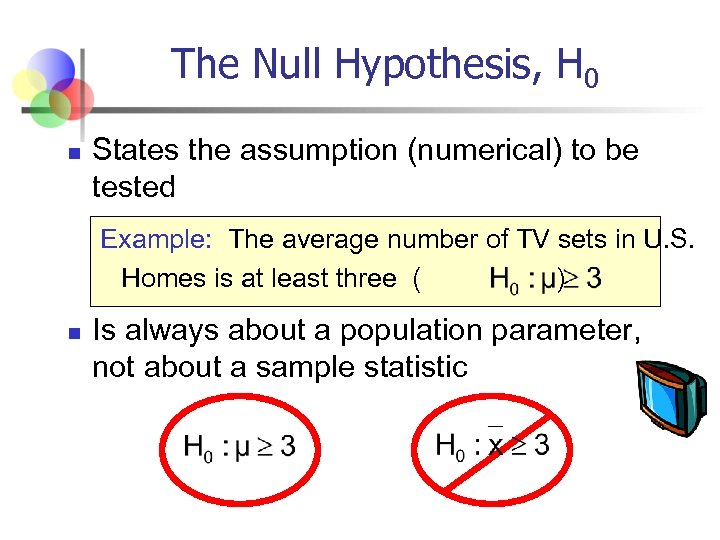The Null Hypothesis, H 0 n States the assumption (numerical) to be tested Example: The average number of TV sets in U. S. Homes is at least three ( ) n Is always about a population parameter, not about a sample statistic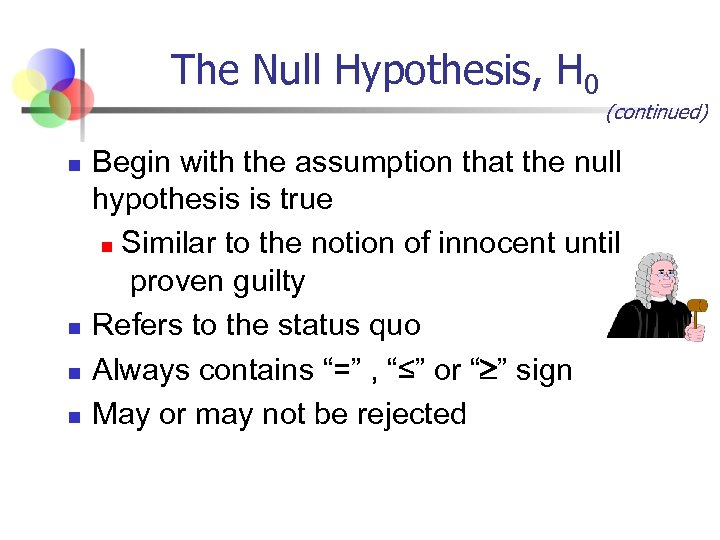The Null Hypothesis, H 0 n n (continued) Begin with the assumption that the null hypothesis is true n Similar to the notion of innocent until proven guilty Refers to the status quo Always contains “=” , “≤” or “ ” sign May or may not be rejected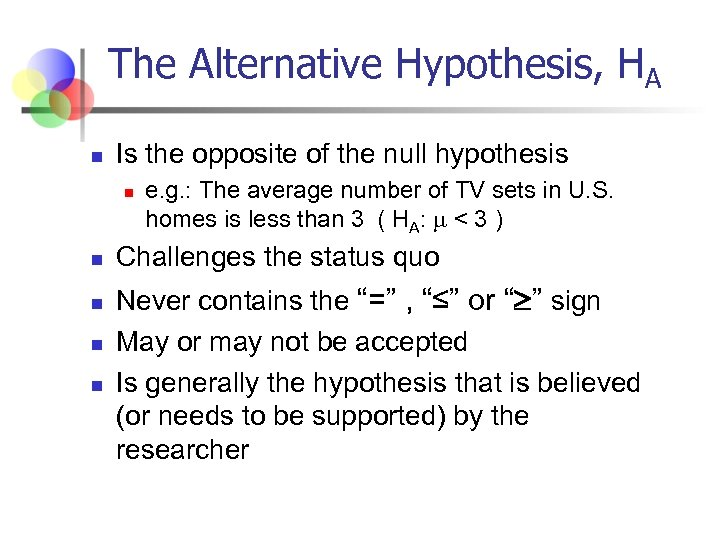The Alternative Hypothesis, HA n Is the opposite of the null hypothesis n n n e. g. : The average number of TV sets in U. S. homes is less than 3 ( HA: < 3 ) Challenges the status quo Never contains the “=” , “≤” or “ ” sign May or may not be accepted Is generally the hypothesis that is believed (or needs to be supported) by the researcher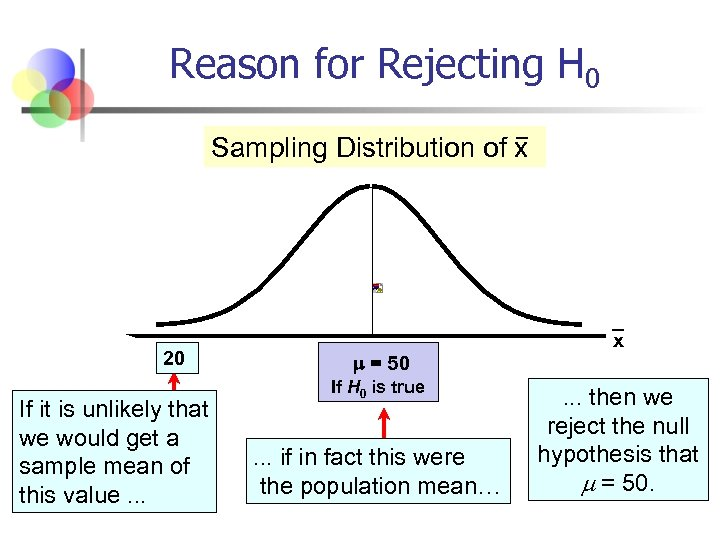Reason for Rejecting H 0 Sampling Distribution of x 20 If it is unlikely that we would get a sample mean of this value. . . = 50 If H 0 is true . . . if in fact this were the population mean… x . . . then we reject the null hypothesis that = 50.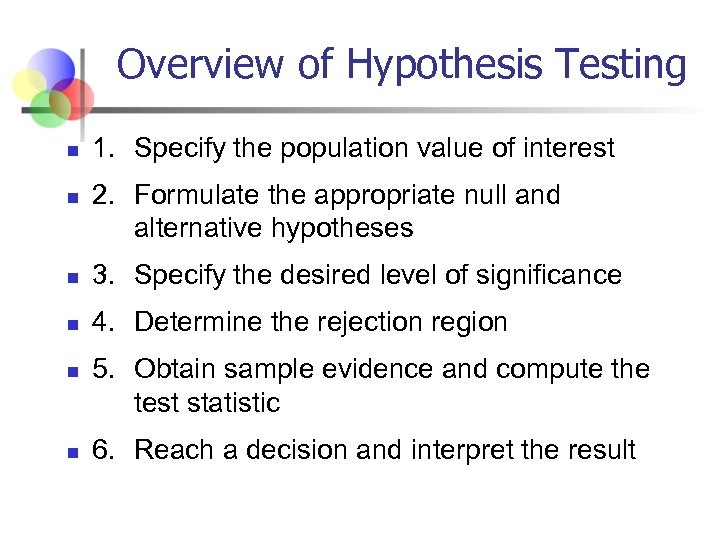Overview of Hypothesis Testing n n 1. Specify the population value of interest 2. Formulate the appropriate null and alternative hypotheses n 3. Specify the desired level of significance n 4. Determine the rejection region n n 5. Obtain sample evidence and compute the test statistic 6. Reach a decision and interpret the result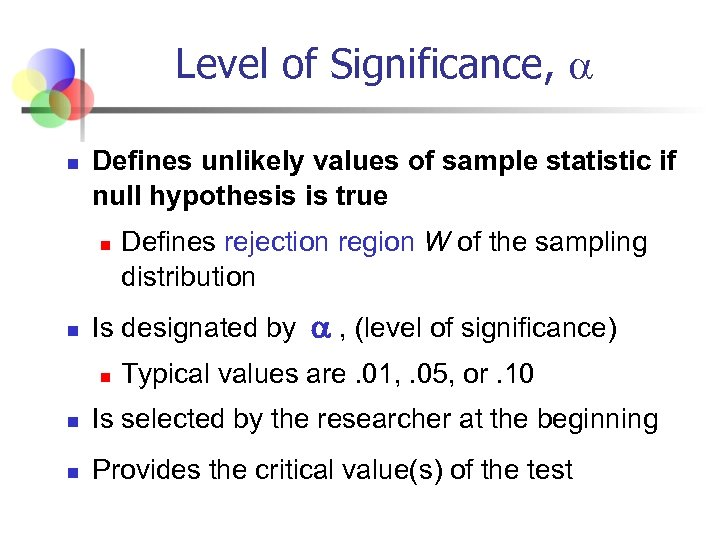Level of Significance, n Defines unlikely values of sample statistic if null hypothesis is true n n Defines rejection region W of the sampling distribution Is designated by , (level of significance) n Typical values are. 01, . 05, or. 10 n Is selected by the researcher at the beginning n Provides the critical value(s) of the test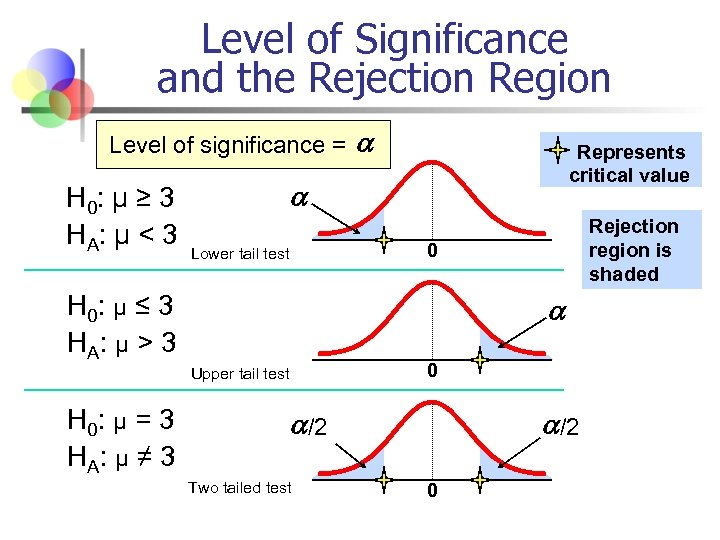Level of Significance and the Rejection Region Level of significance = H 0: μ ≥ 3 HA : μ < 3 Represents critical value Rejection region is shaded 0 Lower tail test H 0: μ ≤ 3 HA : μ > 3 0 Upper tail test H 0: μ = 3 HA : μ ≠ 3 /2 Two tailed test /2 0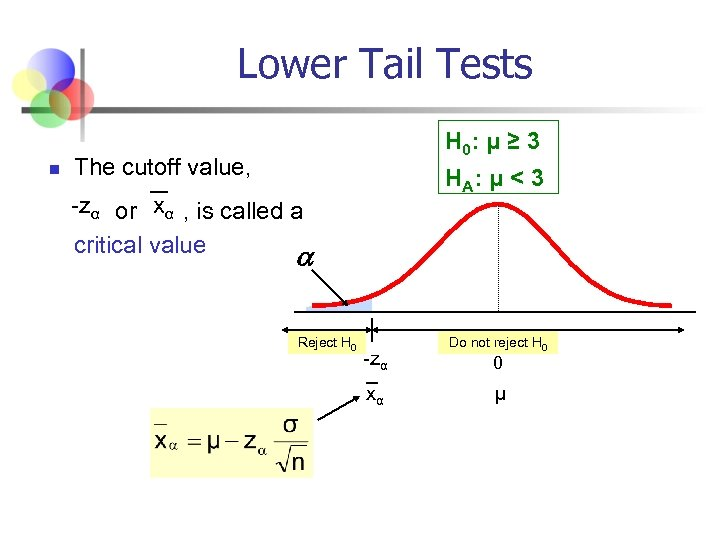Lower Tail Tests n H 0: μ ≥ 3 The cutoff value, H A: μ < 3 -zα or xα , is called a critical value Reject H 0 -zα xα Do not reject H 0 0 μ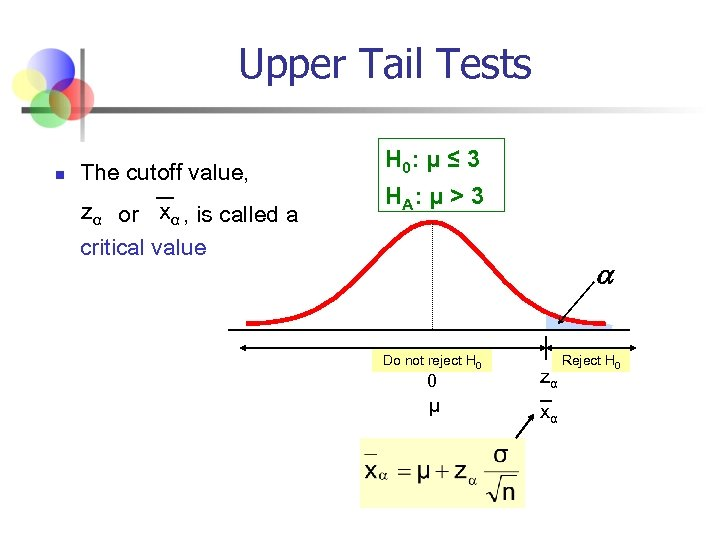Upper Tail Tests n The cutoff value, zα or xα , is called a critical value H 0: μ ≤ 3 H A: μ > 3 Do not reject H 0 0 zα μ xα Reject H 0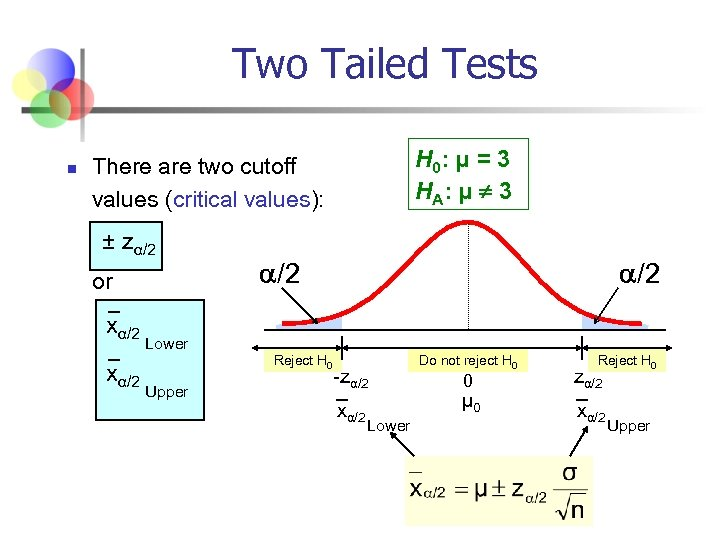Two Tailed Tests n H 0: μ = 3 H A: μ ¹ 3 There are two cutoff values (critical values): ± zα/2 or xα/2 /2 Lower Reject H 0 Upper -zα/2 xα/2 Lower Do not reject H 0 0 μ 0 Reject H 0 zα/2 xα/2 Upper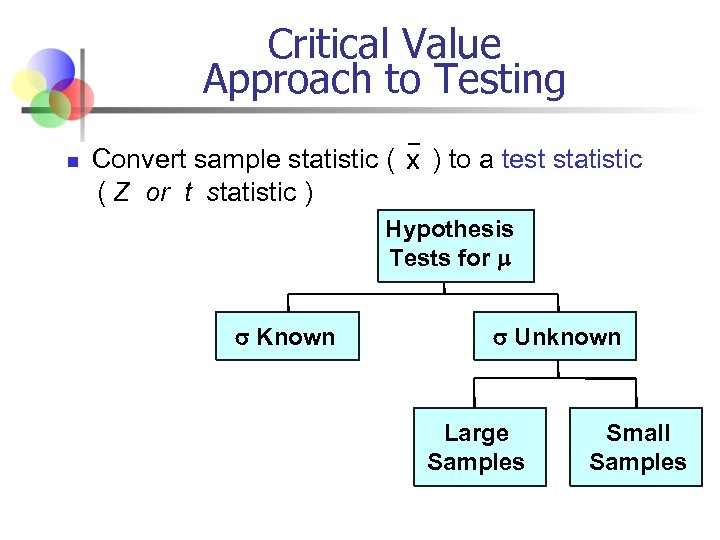Critical Value Approach to Testing n Convert sample statistic ( x ) to a test statistic ( Z or t statistic ) Hypothesis Tests for Known Unknown Large Samples Small Samples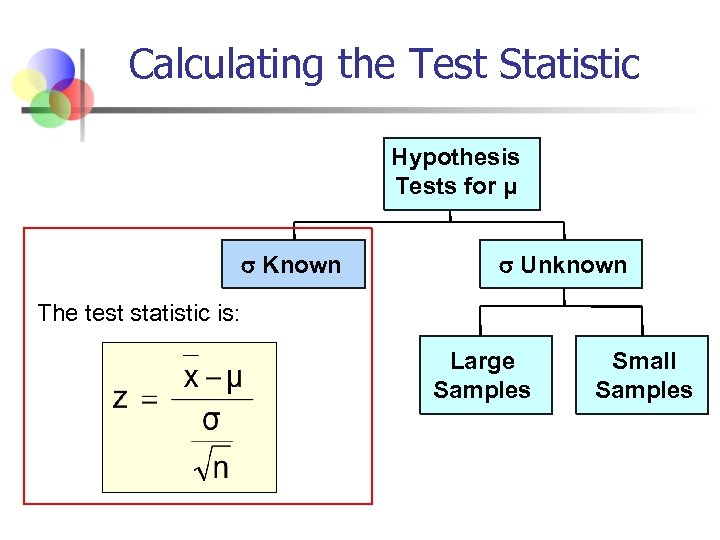Calculating the Test Statistic Hypothesis Tests for μ Known Unknown The test statistic is: Large Samples Small Samples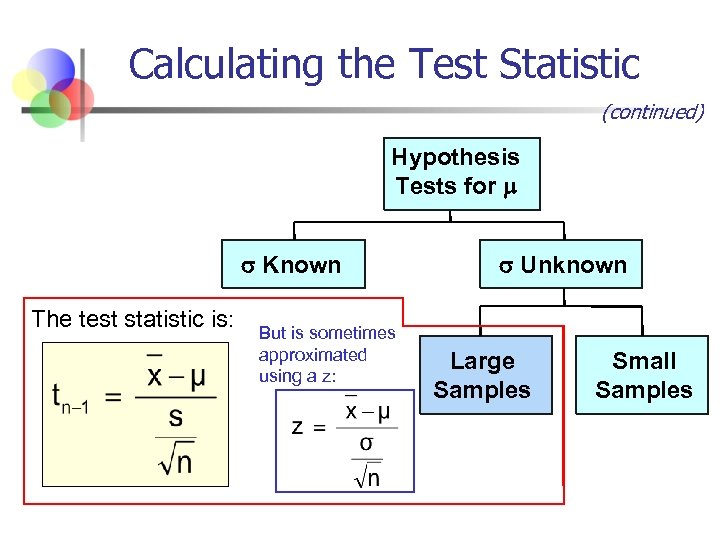Calculating the Test Statistic (continued) Hypothesis Tests for Known The test statistic is: But is sometimes approximated using a z: Unknown Large Samples Small Samples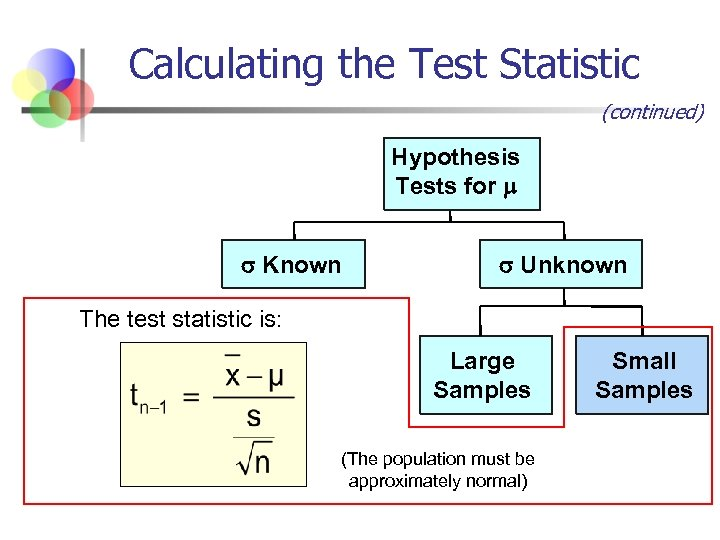Calculating the Test Statistic (continued) Hypothesis Tests for Known Unknown The test statistic is: Large Samples (The population must be approximately normal) Small Samples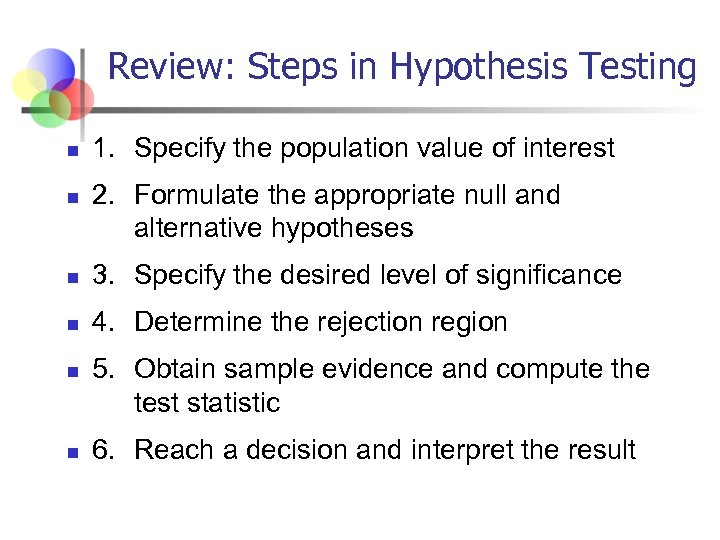Review: Steps in Hypothesis Testing n n 1. Specify the population value of interest 2. Formulate the appropriate null and alternative hypotheses n 3. Specify the desired level of significance n 4. Determine the rejection region n n 5. Obtain sample evidence and compute the test statistic 6. Reach a decision and interpret the result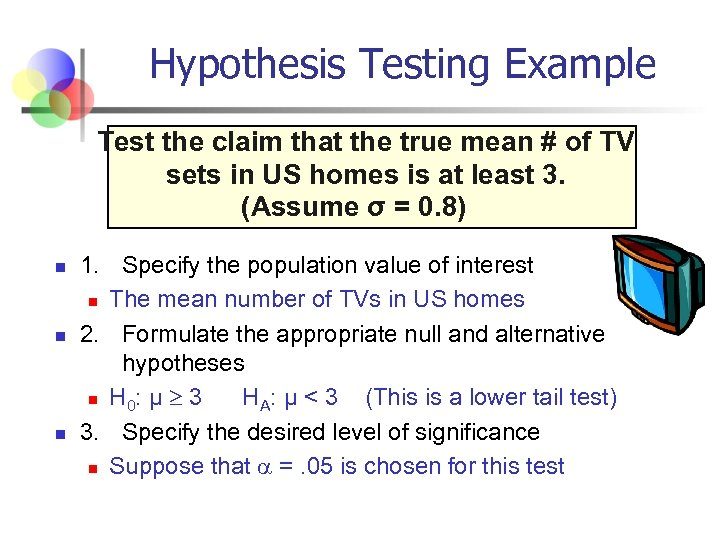Hypothesis Testing Example Test the claim that the true mean # of TV sets in US homes is at least 3. (Assume σ = 0. 8) n n n 1. Specify the population value of interest n The mean number of TVs in US homes 2. Formulate the appropriate null and alternative hypotheses n H 0: μ 3 HA: μ < 3 (This is a lower tail test) 3. Specify the desired level of significance n Suppose that =. 05 is chosen for this test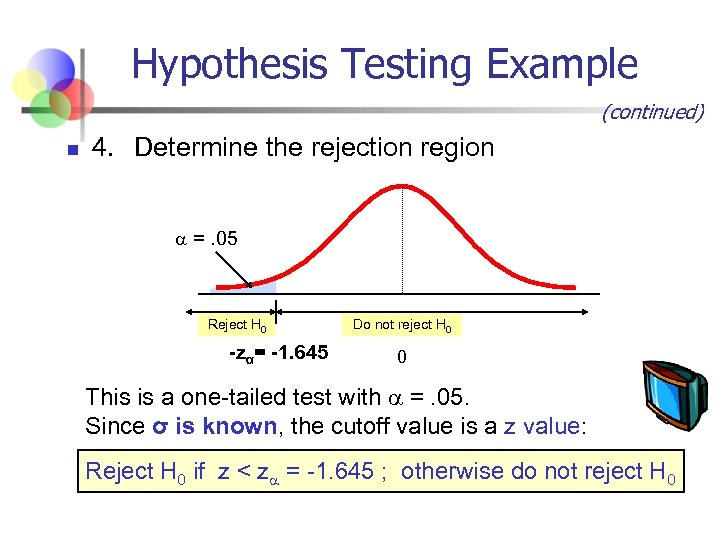Hypothesis Testing Example (continued) n 4. Determine the rejection region =. 05 Reject H 0 -zα= -1. 645 Do not reject H 0 0 This is a one-tailed test with =. 05. Since σ is known, the cutoff value is a z value: Reject H 0 if z < z = -1. 645 ; otherwise do not reject H 0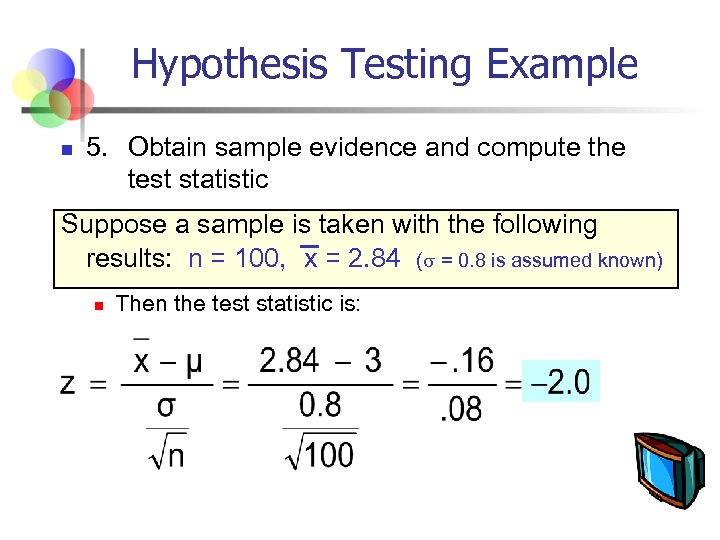Hypothesis Testing Example n 5. Obtain sample evidence and compute the test statistic Suppose a sample is taken with the following results: n = 100, x = 2. 84 ( = 0. 8 is assumed known) n Then the test statistic is: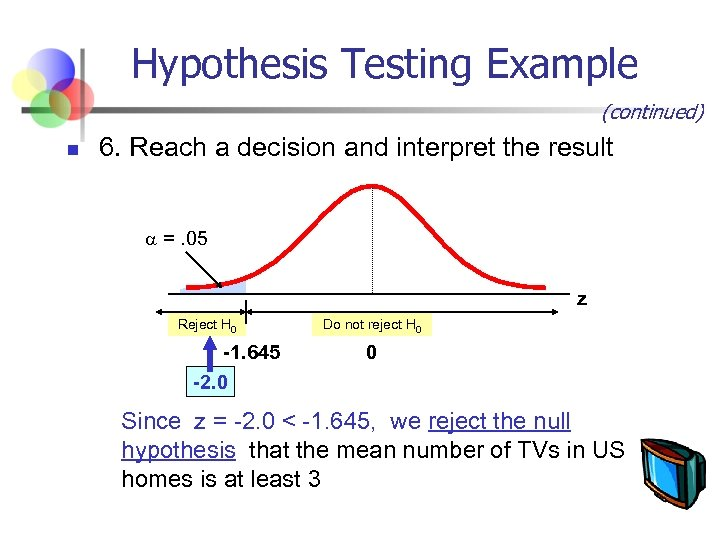Hypothesis Testing Example (continued) n 6. Reach a decision and interpret the result =. 05 z Reject H 0 -1. 645 -2. 0 Do not reject H 0 0 Since z = -2. 0 < -1. 645, we reject the null hypothesis that the mean number of TVs in US homes is at least 3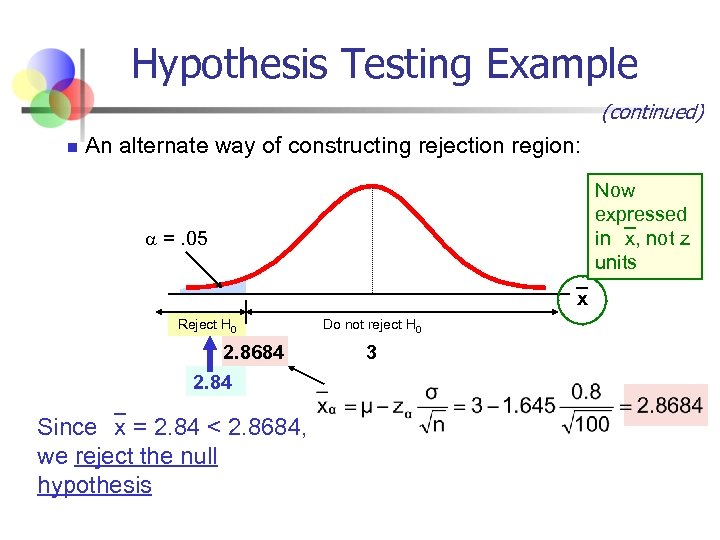Hypothesis Testing Example (continued) n An alternate way of constructing rejection region: Now expressed in x, not z units =. 05 x Reject H 0 2. 8684 2. 84 Since x = 2. 84 < 2. 8684, we reject the null hypothesis Do not reject H 0 3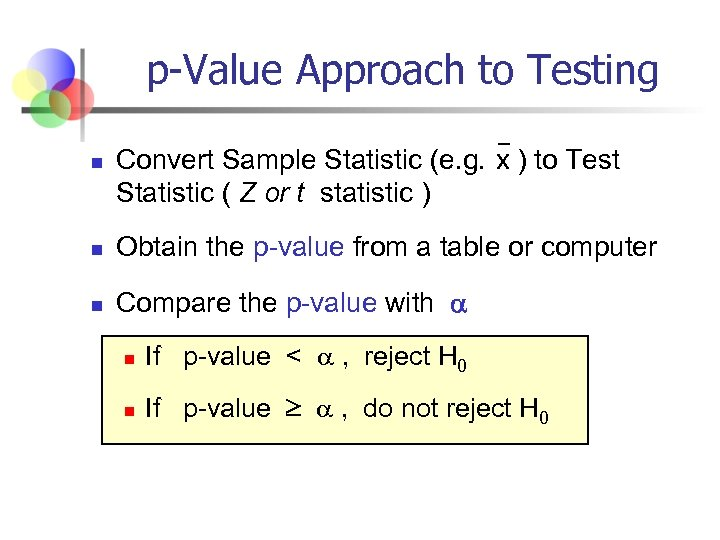p-Value Approach to Testing n Convert Sample Statistic (e. g. x ) to Test Statistic ( Z or t statistic ) n Obtain the p-value from a table or computer n Compare the p-value with n If p-value < , reject H 0 n If p-value , do not reject H 0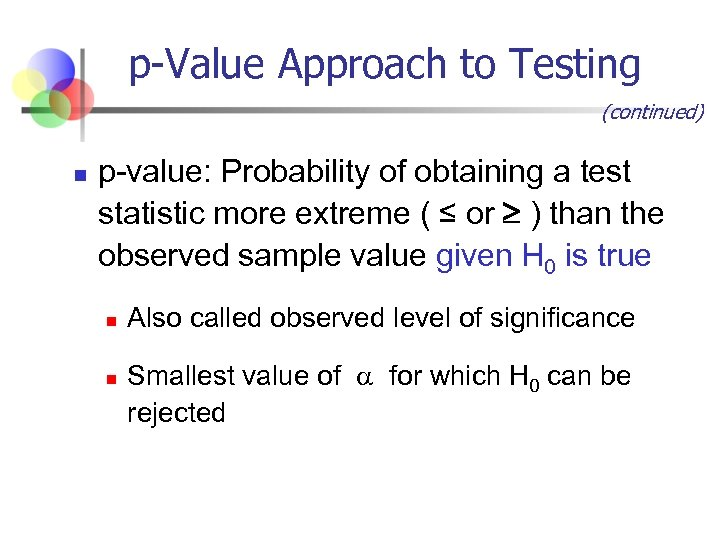p-Value Approach to Testing (continued) n p-value: Probability of obtaining a test statistic more extreme ( ≤ or ) than the observed sample value given H 0 is true n n Also called observed level of significance Smallest value of for which H 0 can be rejected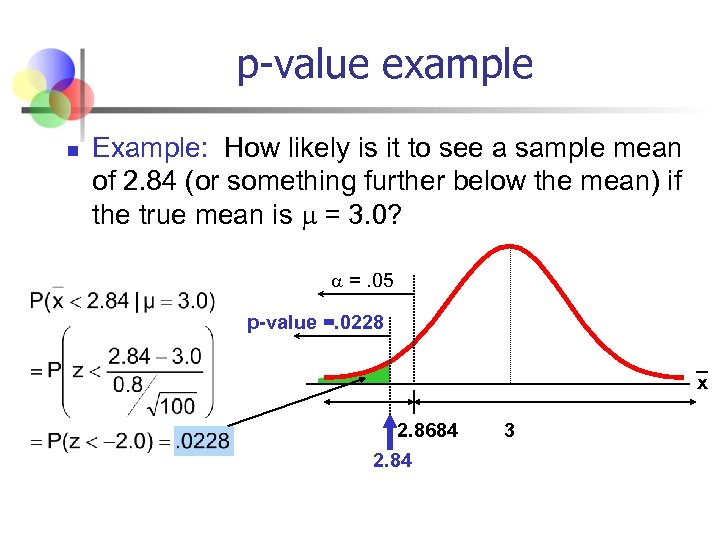p-value example n Example: How likely is it to see a sample mean of 2. 84 (or something further below the mean) if the true mean is = 3. 0? =. 05 p-value =. 0228 x 2. 8684 2. 84 3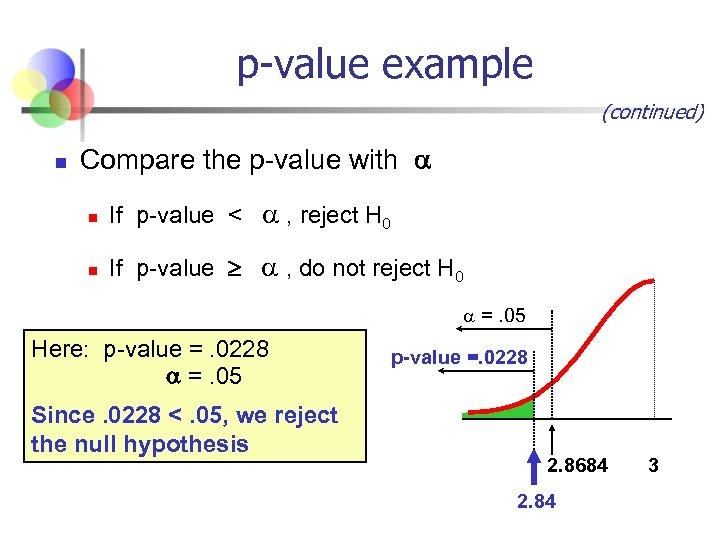p-value example (continued) n Compare the p-value with n If p-value < , reject H 0 n If p-value , do not reject H 0 =. 05 Here: p-value =. 0228 =. 05 Since. 0228 <. 05, we reject the null hypothesis p-value =. 0228 2. 8684 2. 84 3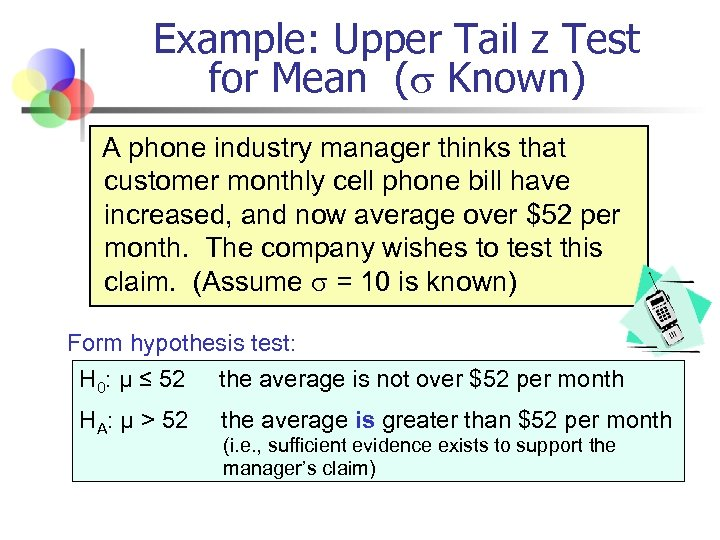Example: Upper Tail z Test for Mean ( Known) A phone industry manager thinks that customer monthly cell phone bill have increased, and now average over \$52 per month. The company wishes to test this claim. (Assume = 10 is known) Form hypothesis test: H 0: μ ≤ 52 the average is not over \$52 per month HA: μ > 52 the average is greater than \$52 per month (i. e. , sufficient evidence exists to support the manager’s claim)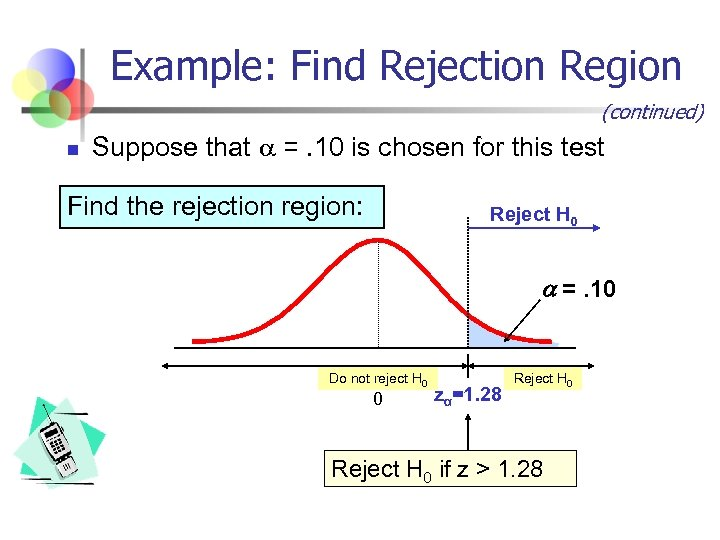Example: Find Rejection Region (continued) n Suppose that =. 10 is chosen for this test Find the rejection region: Reject H 0 =. 10 Do not reject H 0 0 zα=1. 28 Reject H 0 if z > 1. 28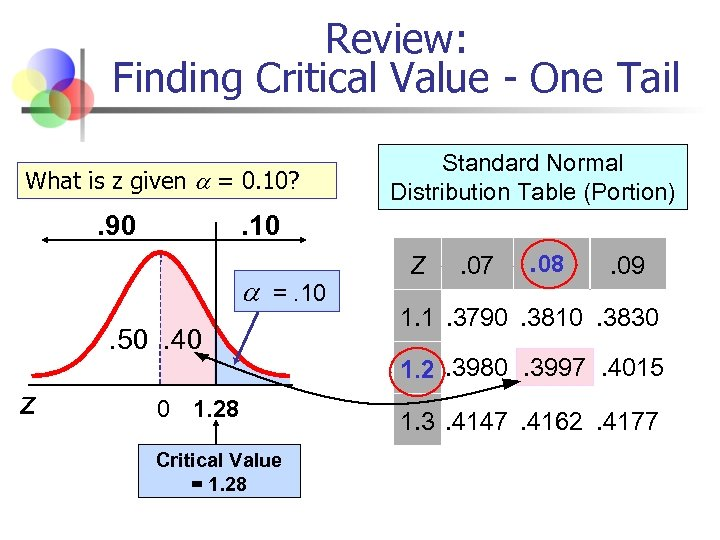Review: Finding Critical Value - One Tail What is z given = 0. 10? . 90 . 10 =. 10. 50. 40 z Standard Normal Distribution Table (Portion) 0 1. 28 Critical Value = 1. 28 Z . 07 . 08 . 09 1. 1. 3790. 3810. 3830 1. 2. 3980. 3997. 4015 1. 3. 4147. 4162. 4177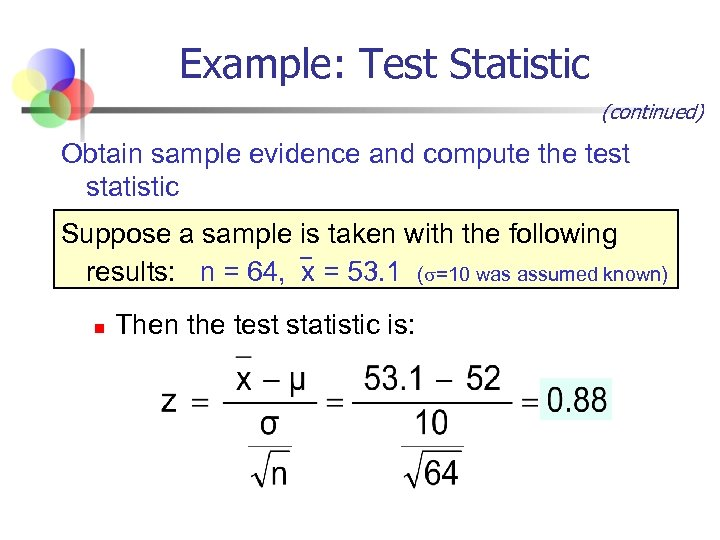Example: Test Statistic (continued) Obtain sample evidence and compute the test statistic Suppose a sample is taken with the following results: n = 64, x = 53. 1 ( =10 was assumed known) n Then the test statistic is: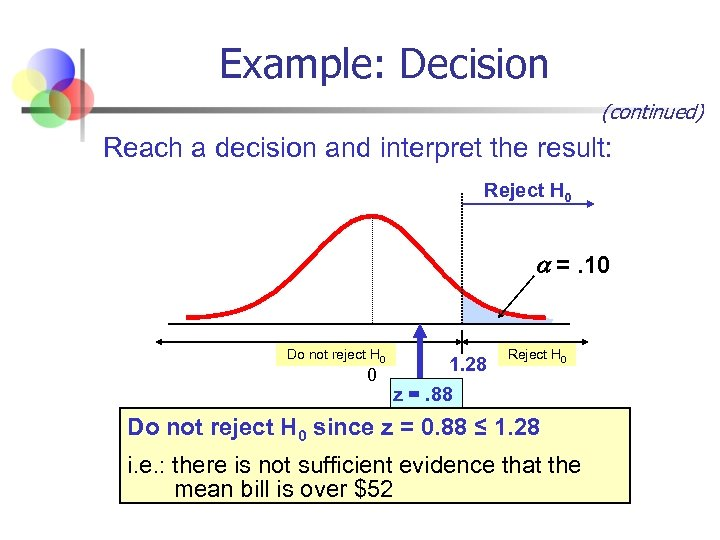Example: Decision (continued) Reach a decision and interpret the result: Reject H 0 =. 10 Do not reject H 0 1. 28 0 z =. 88 Reject H 0 Do not reject H 0 since z = 0. 88 ≤ 1. 28 i. e. : there is not sufficient evidence that the mean bill is over \$52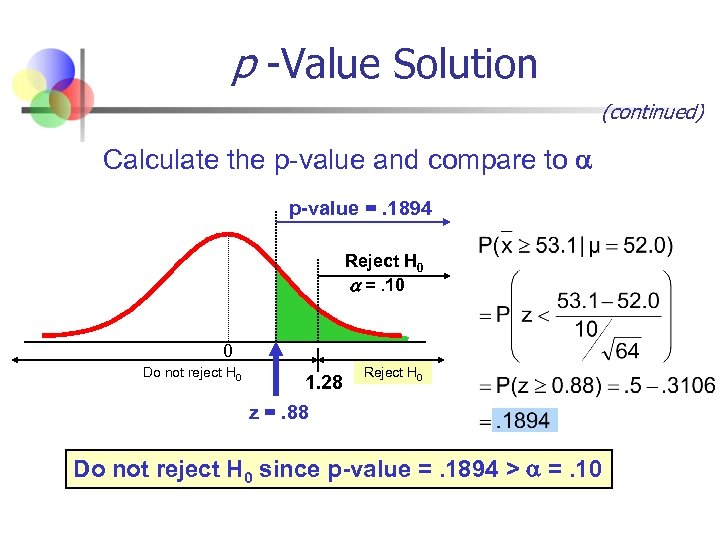p -Value Solution (continued) Calculate the p-value and compare to p-value =. 1894 Reject H 0 =. 10 0 Do not reject H 0 1. 28 z =. 88 Reject H 0 Do not reject H 0 since p-value =. 1894 > =. 10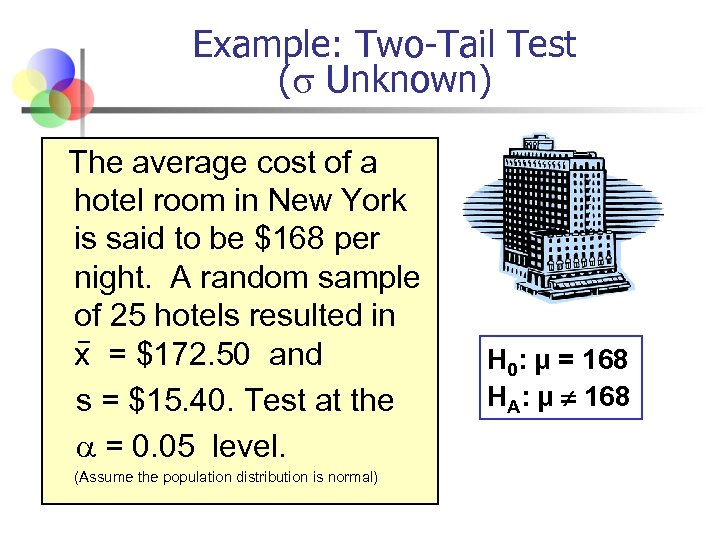Example: Two-Tail Test ( Unknown) The average cost of a hotel room in New York is said to be \$168 per night. A random sample of 25 hotels resulted in x = \$172. 50 and s = \$15. 40. Test at the = 0. 05 level. (Assume the population distribution is normal) H 0: μ = 168 HA: μ ¹ 168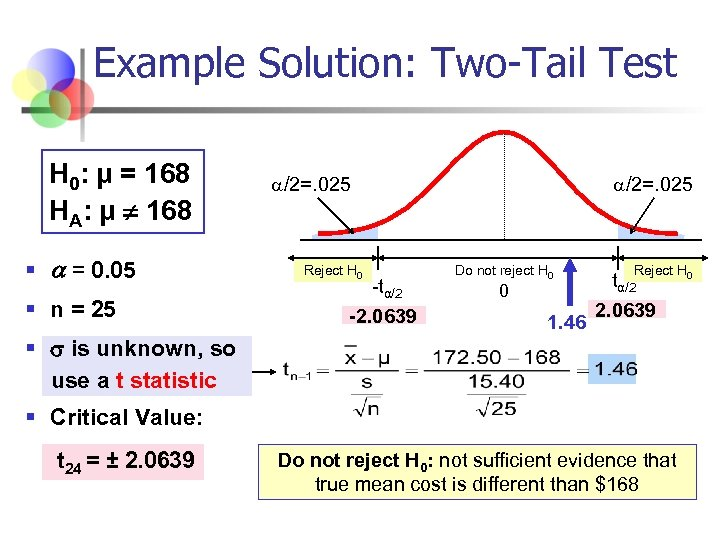Example Solution: Two-Tail Test H 0: μ = 168 HA: μ ¹ 168 § = 0. 05 § n = 25 § is unknown, so use a t statistic /2=. 025 Reject H 0 -tα/2 -2. 0639 /2=. 025 Do not reject H 0 0 1. 46 Reject H 0 tα/2 2. 0639 § Critical Value: t 24 = ± 2. 0639 Do not reject H 0: not sufficient evidence that true mean cost is different than \$168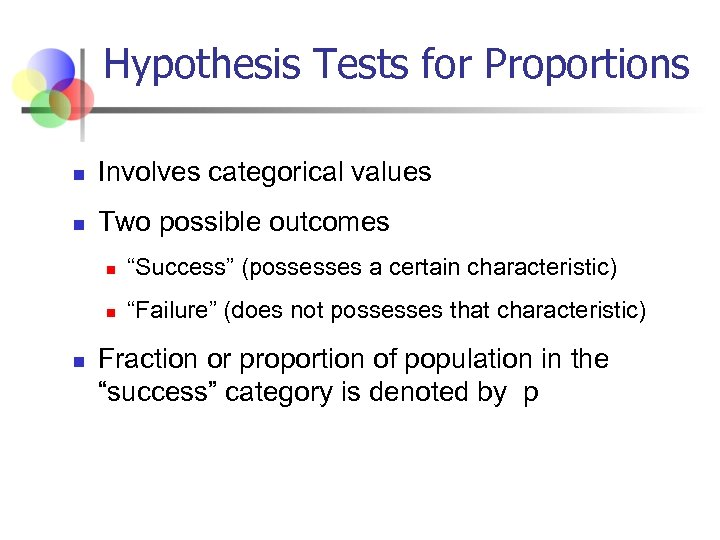Hypothesis Tests for Proportions n Involves categorical values n Two possible outcomes n n n “Success” (possesses a certain characteristic) “Failure” (does not possesses that characteristic) Fraction or proportion of population in the “success” category is denoted by p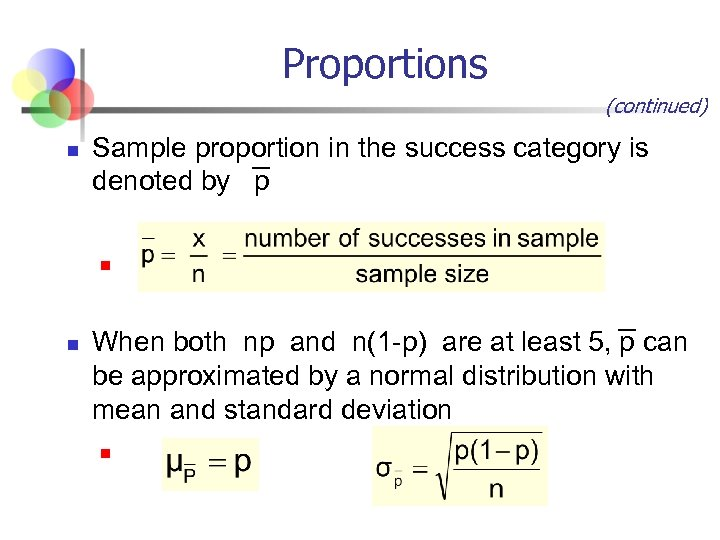Proportions (continued) n Sample proportion in the success category is denoted by p n n When both np and n(1 -p) are at least 5, p can be approximated by a normal distribution with mean and standard deviation n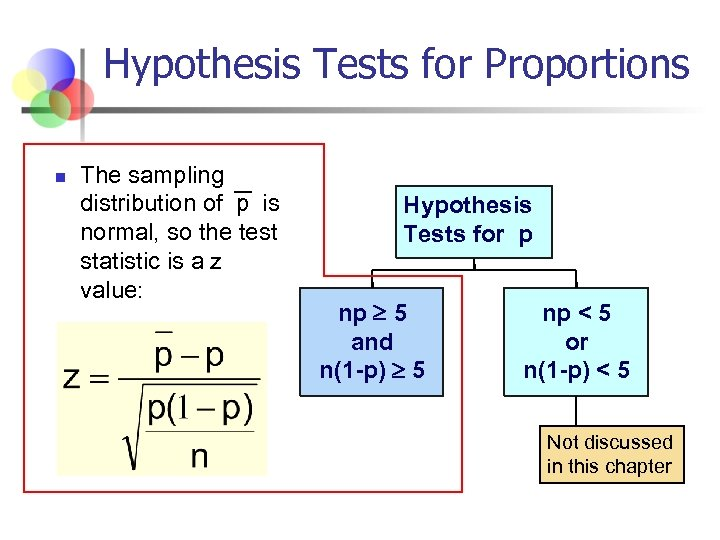Hypothesis Tests for Proportions n The sampling distribution of p is normal, so the test statistic is a z value: Hypothesis Tests for p np 5 and n(1 -p) 5 np < 5 or n(1 -p) < 5 Not discussed in this chapter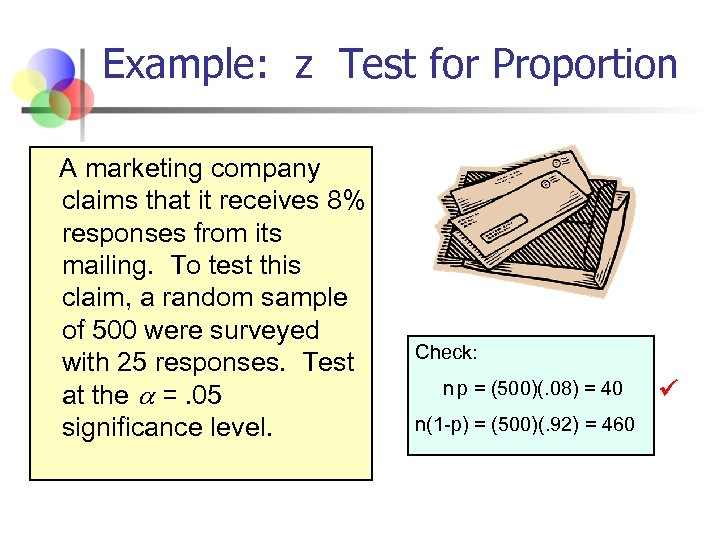Example: z Test for Proportion A marketing company claims that it receives 8% responses from its mailing. To test this claim, a random sample of 500 were surveyed with 25 responses. Test at the =. 05 significance level. Check: n p = (500)(. 08) = 40 n(1 -p) = (500)(. 92) = 460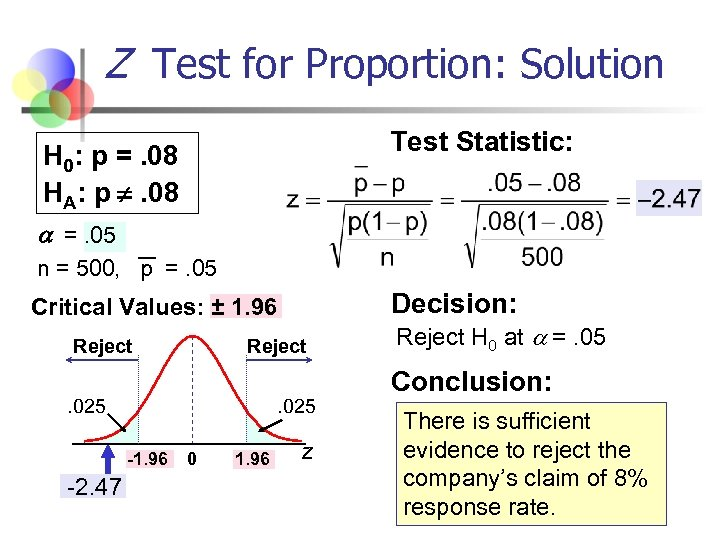Z Test for Proportion: Solution Test Statistic: H 0: p =. 08 HA: p ¹. 08 =. 05 n = 500, p =. 05 Decision: Critical Values: ± 1. 96 Reject . 025 -1. 96 -2. 47 0 1. 96 z Reject H 0 at =. 05 Conclusion: There is sufficient evidence to reject the company’s claim of 8% response rate.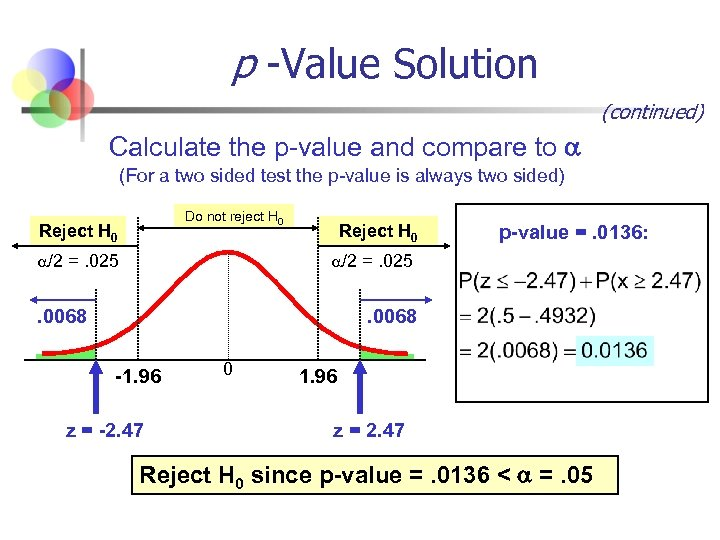p -Value Solution (continued) Calculate the p-value and compare to (For a two sided test the p-value is always two sided) Do not reject H 0 Reject H 0 /2 =. 025 Reject H 0 p-value =. 0136: /2 =. 025 . 0068 -1. 96 z = -2. 47 0 1. 96 z = 2. 47 Reject H 0 since p-value =. 0136 < =. 05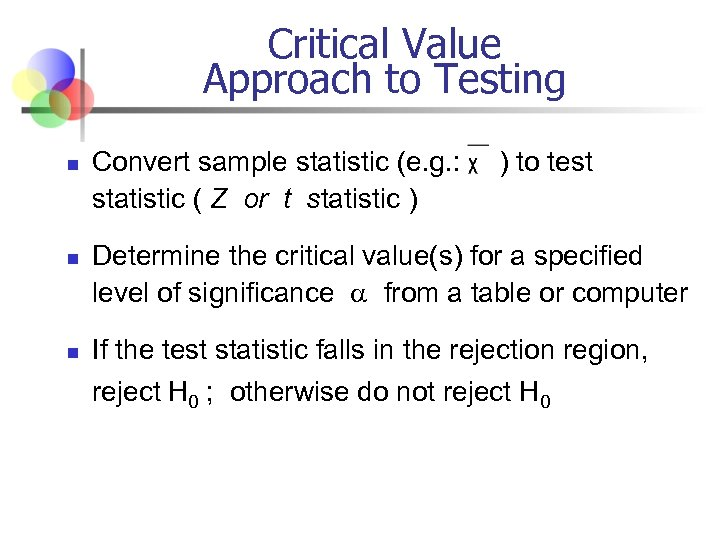Critical Value Approach to Testing n n n Convert sample statistic (e. g. : statistic ( Z or t statistic ) ) to test Determine the critical value(s) for a specified level of significance from a table or computer If the test statistic falls in the rejection region, reject H 0 ; otherwise do not reject H 0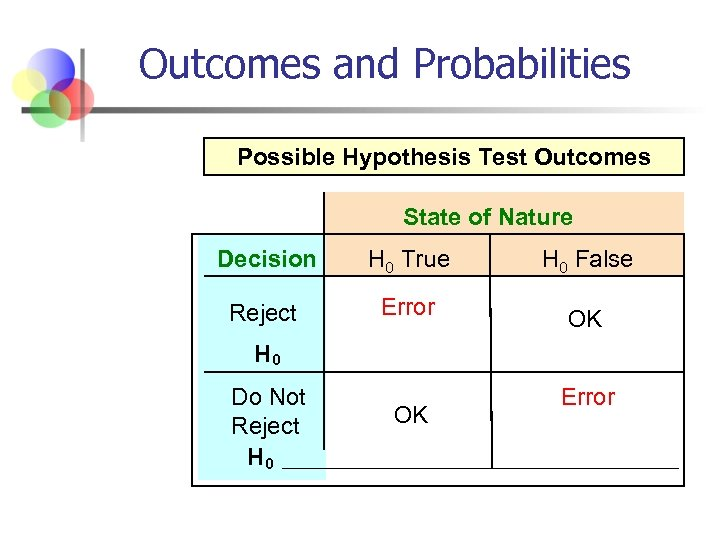Outcomes and Probabilities Possible Hypothesis Test Outcomes State of Nature Decision H 0 True H 0 False Reject Error OK H 0 Do Not Reject H 0 OK Error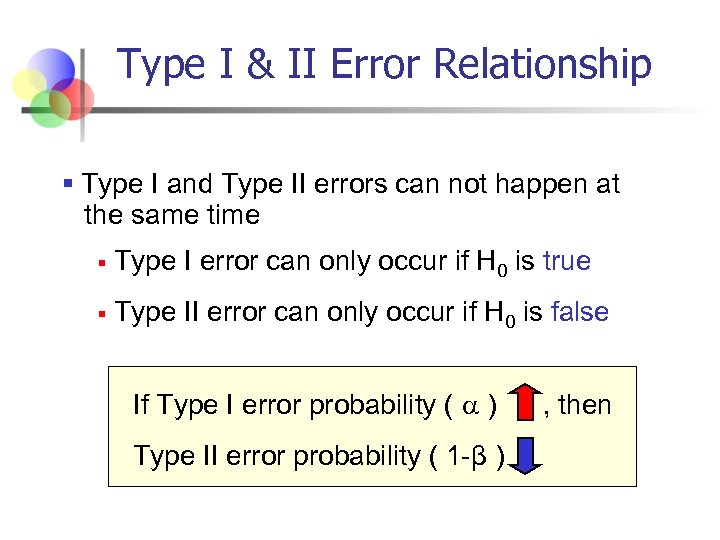Type I & II Error Relationship § Type I and Type II errors can not happen at the same time § Type I error can only occur if H 0 is true § Type II error can only occur if H 0 is false If Type I error probability ( ) Type II error probability ( 1 -β ) , then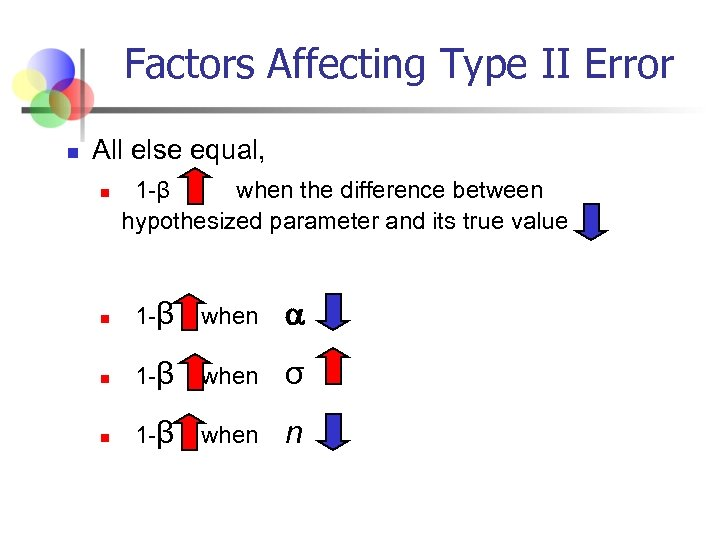Factors Affecting Type II Error n All else equal, n 1 -β when the difference between hypothesized parameter and its true value n 1 -β when σ n 1 -β when n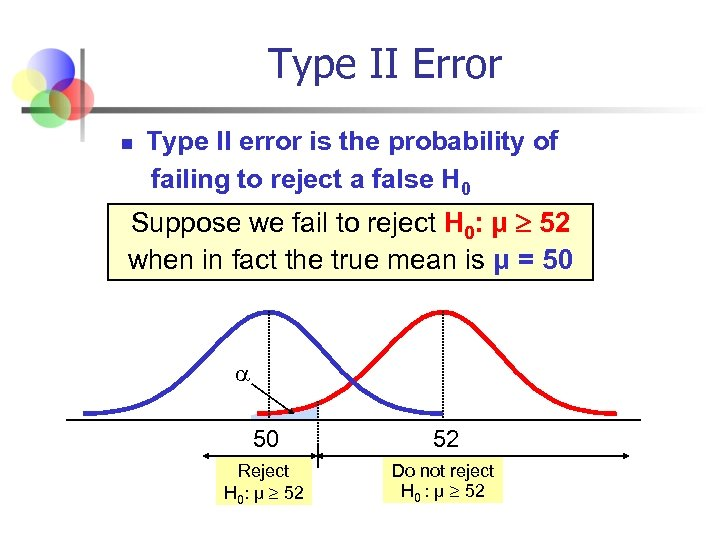Type II Error n Type II error is the probability of failing to reject a false H 0 Suppose we fail to reject H 0: μ 52 when in fact the true mean is μ = 50 52 Reject H 0: μ 52 Do not reject H 0 : μ 52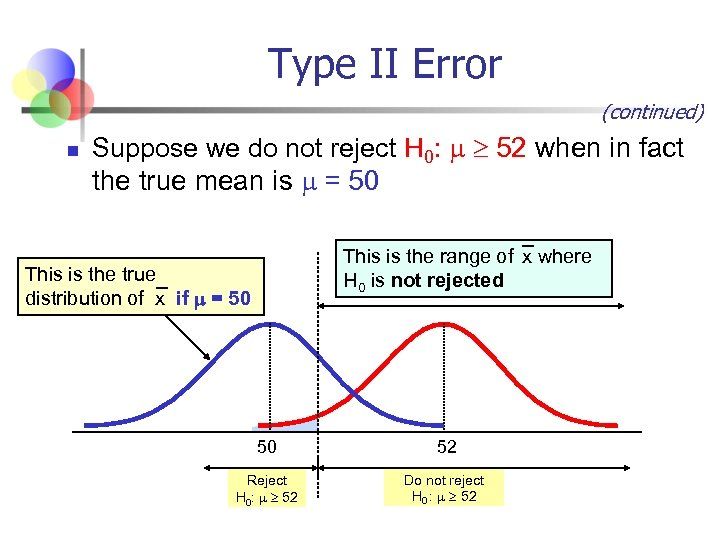Type II Error (continued) n Suppose we do not reject H 0: 52 when in fact the true mean is = 50 This is the range of x where H 0 is not rejected This is the true distribution of x if = 50 50 52 Reject H 0: 52 Do not reject H 0 : 52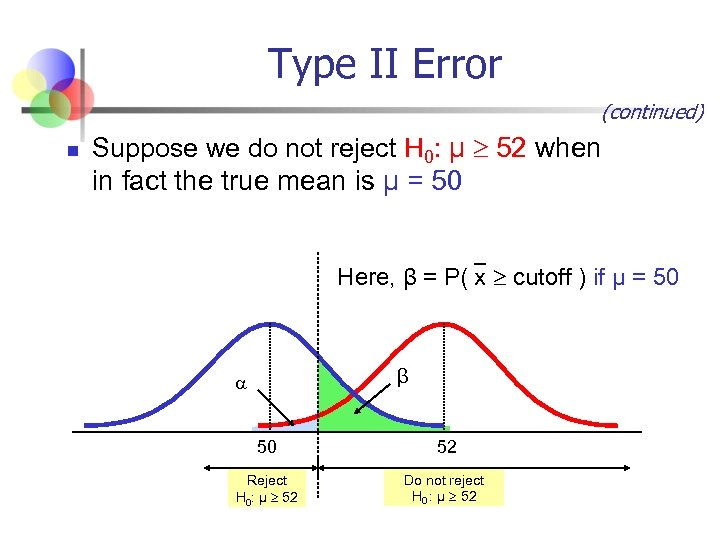Type II Error (continued) n Suppose we do not reject H 0: μ 52 when in fact the true mean is μ = 50 Here, β = P( x cutoff ) if μ = 50 β 50 52 Reject H 0: μ 52 Do not reject H 0 : μ 52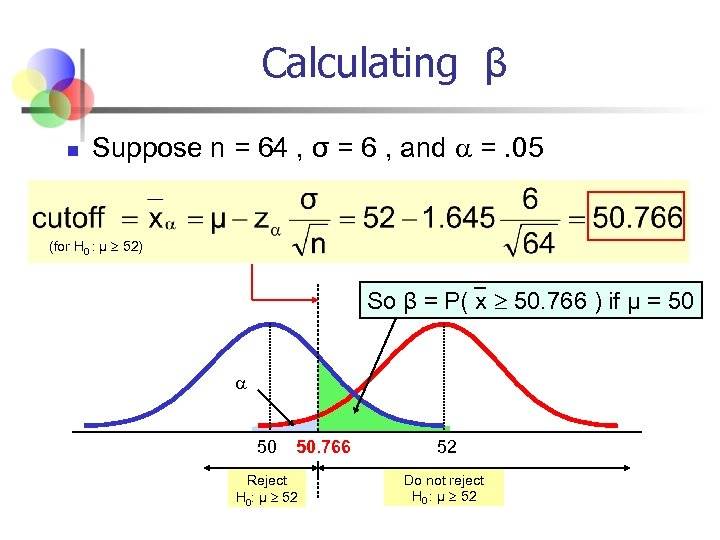Calculating β n Suppose n = 64 , σ = 6 , and =. 05 (for H 0 : μ 52) So β = P( x 50. 766 ) if μ = 50 50. 766 Reject H 0: μ 52 52 Do not reject H 0 : μ 52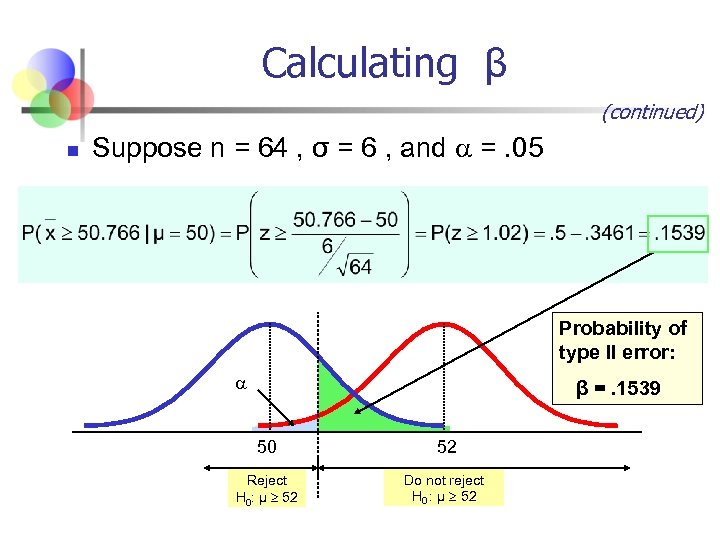Calculating β (continued) n Suppose n = 64 , σ = 6 , and =. 05 Probability of type II error: β =. 1539 50 52 Reject H 0: μ 52 Do not reject H 0 : μ 52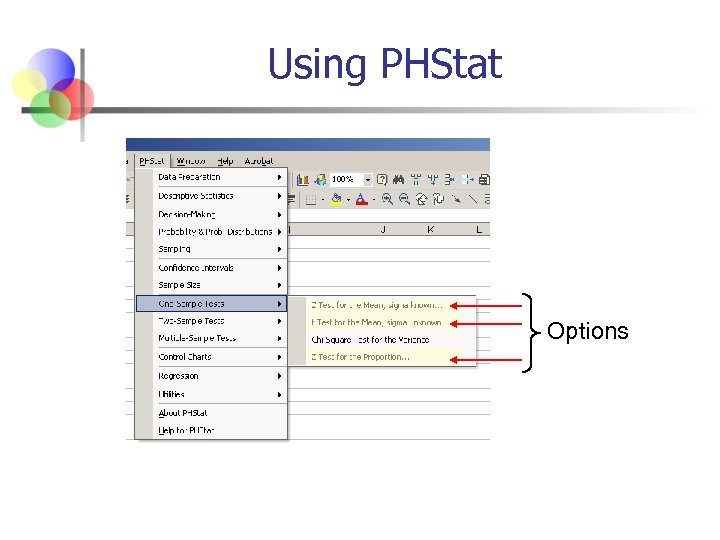Using PHStat Options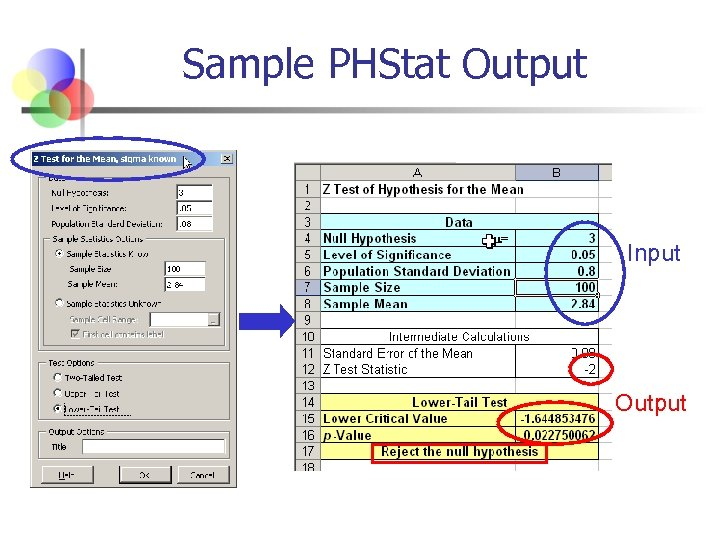Sample PHStat Output Input Output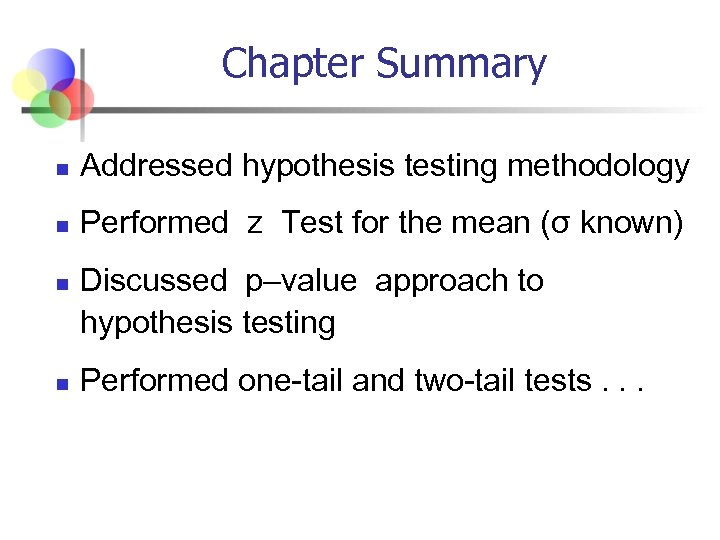Chapter Summary n Addressed hypothesis testing methodology n Performed z Test for the mean (σ known) n n Discussed p–value approach to hypothesis testing Performed one-tail and two-tail tests. . .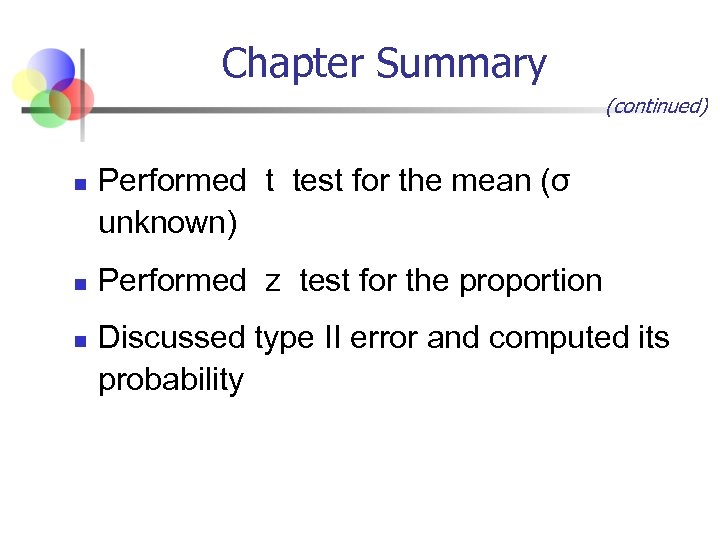Chapter Summary (continued) n n n Performed t test for the mean (σ unknown) Performed z test for the proportion Discussed type II error and computed its probability